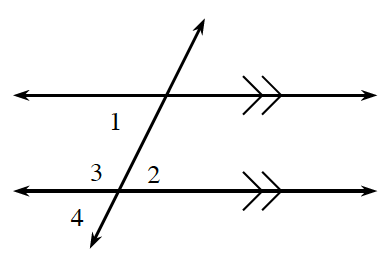### Home > CC1MN > Chapter 10 > Lesson 10.3.1 > Problem10-73

10-73.

Use the conjectures and definitions in this lesson to solve parts (a) and (b). Each part is a separate problem.

1. If $m \angle 1 = 63º$, find $m\angle 2$ and $m\angle 3$ by calculation.

Angle 1 and angle 2 are alternate interior angles.

When the lines are parallel, alternate interior angles have equal measures.

2. If $m \angle 1 = 74º$ and $m \angle 4 = 3x-18º$, write an equation and find $x$.

Disregard your calculations for angle measure from part (a).

$3x − 18 = 74$

$3x = 92$

$x = 30.6°$

3. If $m \angle 2 = 3x-9º$ and $m \angle 1 = x + 25º$, write an equation to find $x$. Then find $m\angle 2$.

Since the lines are parallel, angles 1 and 2 have equal measure.

$x = 17º, m∠2 = 42º$